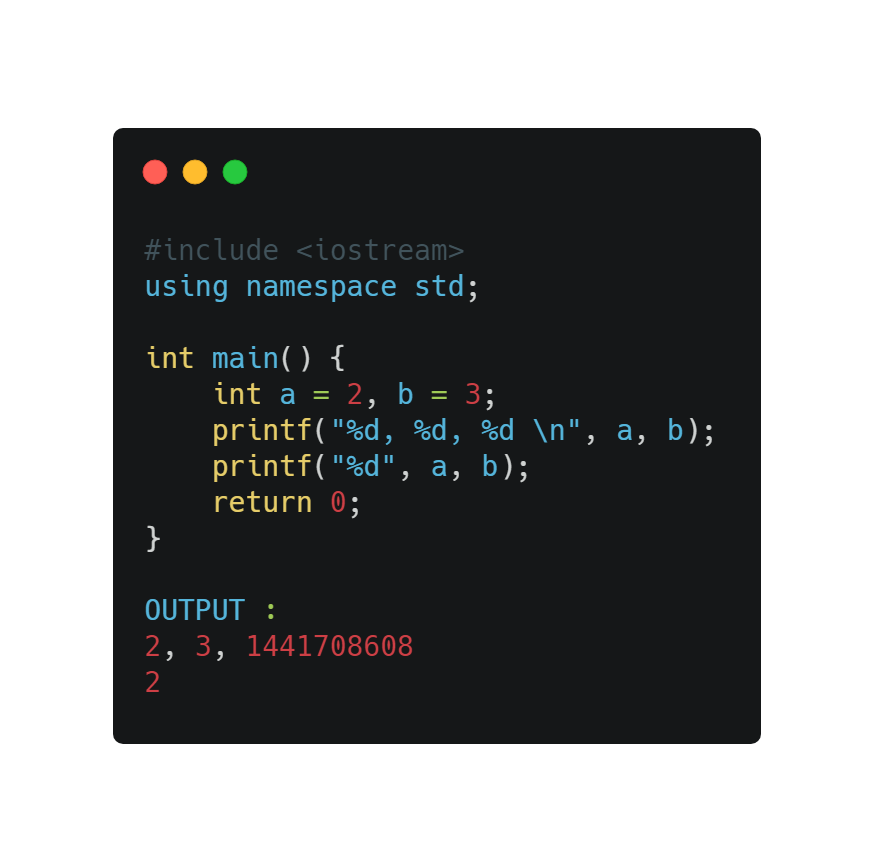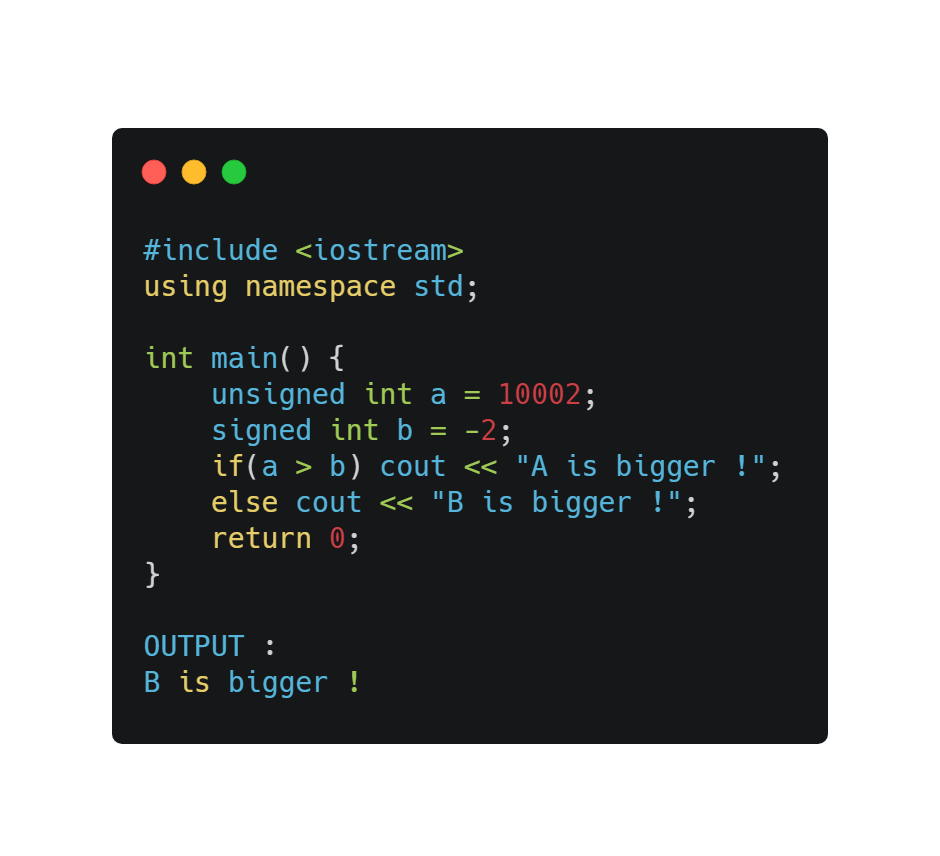1590609660

# Count of triplets (a, b, c) in the Array such that a divides b and b divides c

Given an array arr[] of positive integers of size N, the task is to count number of triplets in the array such that a[i] divides a[j] and a[j] divides a[k] and i < j < k.

Examples:

``````Input: arr[] = {1, 2, 3, 4, 5, 6}
Output: 3
Explanation:
The triplets are: (1, 2, 4), (1, 2, 6), (1, 3, 6).``````

#c #c# #c++ #programming-c

## Buddha Community1590609660

## Count of triplets (a, b, c) in the Array such that a divides b and b divides c

Given an array arr[] of positive integers of size N, the task is to count number of triplets in the array such that a[i] divides a[j] and a[j] divides a[k] and i < j < k.

Examples:

``````Input: arr[] = {1, 2, 3, 4, 5, 6}
Output: 3
Explanation:
The triplets are: (1, 2, 4), (1, 2, 6), (1, 3, 6).``````

#c #c# #c++ #programming-c1590293760

## C++ Arrays And Multidimensional Arrays

In this video we are going to talk about C++ Arrays And Multidimensional Arrays, so c++ arrays are collection of the elements that are the same type. if you remember our video on variables, when we want to store one value we are going to use variable. If we want to store two or three values we need to use more variables. but it is not a good way, by this reason we have arrays. also the elements of array are stored in the continues memory location.

#c #c# #c++ #programming-c1590149040

## C++ Arrays And Multidimensional Arrays

In this article we are going to talk about C++ Arrays And Multidimensional Arrays, so c++ arrays are collection of the elements that are the same type. if you remember our article on variables, when we want to store one value we are going to use variable. If we want to store two or three values we need to use more variables. but it is not a good way, by this reason we have arrays. also the elements of array are stored in the continues memory location.

#c++ #c++ tutorials #c #c#1624240146

## How to Run C/C++ in Sublime Text?

C and C++ are the most powerful programming language in the world. Most of the super fast and complex libraries and algorithms are written in C or C++. Most powerful Kernel programs are also written in C. So, there is no way to skip it.

In programming competitions, most programmers prefer to write code in C or C++. Tourist is considered the worlds top programming contestant of all ages who write code in C++.

During programming competitions, programmers prefer to use a lightweight editor to focus on coding and algorithm designing. VimSublime Text, and Notepad++ are the most common editors for us. Apart from the competition, many software developers and professionals love to use Sublime Text just because of its flexibility.

I have discussed the steps we need to complete in this blog post before running a C/C++ code in Sublime Text. We will take the inputs from an input file and print outputs to an output file without using `freopen` file related functions in C/C++.

#cpp #c #c-programming #sublimetext #c++ #c/c++1597937354

## Dicey Issues in C/C++

If you are familiar with C/C++then you must have come across some unusual things and if you haven’t, then you are about to. The below codes are checked twice before adding, so feel free to share this article with your friends. The following displays some of the issues:

1. Using multiple variables in the print function
2. Comparing Signed integer with unsigned integer
3. Putting a semicolon at the end of the loop statement
4. C preprocessor doesn’t need a semicolon
5. Size of the string matters
6. Macros and equations aren’t good friends
7. Never compare Floating data type with double data type
8. Arrays have a boundary
9. Character constants are different from string literals
10. Difference between single(=) and double(==) equal signs.

The below code generates no error since a print function can take any number of inputs but creates a mismatch with the variables. The print function is used to display characters, strings, integers, float, octal, and hexadecimal values onto the output screen. The format specifier is used to display the value of a variable.

1. %d indicates Integer Format Specifier
2. %f indicates Float Format Specifier
3. %c indicates Character Format Specifier
4. %s indicates String Format Specifier
5. %u indicates Unsigned Integer Format Specifier
6. %ld indicates Long Int Format SpecifierA signed integer is a 32-bit datum that encodes an integer in the range [-2147483648 to 2147483647]. An unsigned integer is a 32-bit datum that encodes a non-negative integer in the range [0 to 4294967295]. The signed integer is represented in twos-complement notation. In the below code the signed integer will be converted to the maximum unsigned integer then compared with the unsigned integer.#problems-with-c #dicey-issues-in-c #c-programming #c++ #c #cplusplus